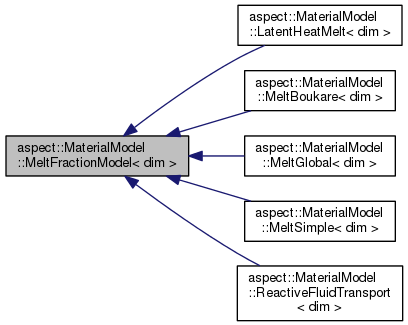ASPECT
aspect::MaterialModel::MeltFractionModel< dim > Class Template Referenceabstract
Inheritance diagram for aspect::MaterialModel::MeltFractionModel< dim >:[legend]

## Public Member Functions

virtual void melt_fractions (const MaterialModel::MaterialModelInputs< dim > &in, std::vector< double > &melt_fractions) const =0

virtual ~MeltFractionModel ()=default

## Detailed Description

### template<int dim> class aspect::MaterialModel::MeltFractionModel< dim >

Base class for material models that implement a melt fraction function. This is used to compute some statistics about the melt fraction.

Definition at line 134 of file melt.h.

## § ~MeltFractionModel()

template<int dim>
 virtual aspect::MaterialModel::MeltFractionModel< dim >::~MeltFractionModel ( )
virtualdefault

Destructor. Does nothing but is virtual so that derived classes destructors are also virtual.

## § melt_fractions()

template<int dim>
 virtual void aspect::MaterialModel::MeltFractionModel< dim >::melt_fractions ( const MaterialModel::MaterialModelInputs< dim > & in, std::vector< double > & melt_fractions ) const
pure virtual

Compute the equilibrium melt fractions for the given input conditions. in and melt_fractions need to have the same size.

Parameters
 in Object that contains the current conditions. melt_fractions Vector of doubles that is filled with the equilibrium melt fraction for each given input conditions.

The documentation for this class was generated from the following file:
• /home/bob/source/include/aspect/melt.h